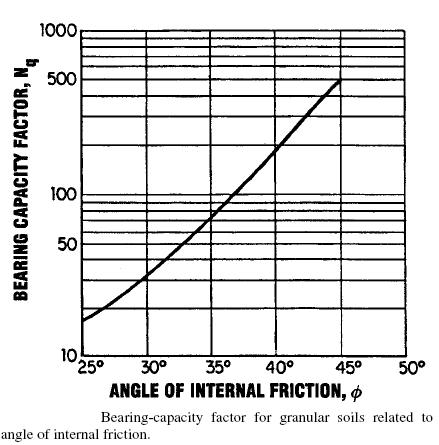Search

Posted in Piles Engineering |Email This Post |

In case of piles being driven in cohesive soils, the ultimate load is alculated by using the followinf formula

Qbu=Abq=AbNccu

where
Ab = End bearing area of pile
q= bearing capacity of soil
Nt =bearing capacity factor
cu= Un-drained shear strength of soil within zone 1 pile diameter above and 2 diameters below pile tip

The value of Nc varies from 8-12 but normally we take the value to by 9

For Cohesionless soils
In case of cohesionless soils, the toe resistance q is calculated as
q=NNq (sigma)’vo<=qt

For Piles driven in Sand
ql=0.5Nq tan(phi)

where
phi= friction angle of the bearing soils below the critical depth.More Entries :